## Ford Fulkerson Algorithm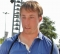Updated on 16-Jun-2020 14:01:32

3K+ Views

The Ford-Fulkerson algorithm is used to detect maximum flow from start vertex to sink vertex in a given graph. In this graph, every edge has the capacity. Two vertices are provided named Source and Sink. The source vertex has all outward edge, no inward edge, and the sink will have all inward edge no outward edge.There are some constraints:Flow on an edge doesn’t exceed the given capacity of that graph.Incoming flow and outgoing flow will also equal for every edge, except the source and the sink.Input and OutputInput: The adjacency matrix: 0 10 0 10 0  0 0  0 4 ... Read More

## Topological Sorting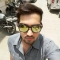Updated on 16-Jun-2020 14:05:13

5K+ Views

The topological sorting for a directed acyclic graph is the linear ordering of vertices. For every edge U-V of a directed graph, the vertex u will come before vertex v in the ordering.As we know that the source vertex will come after the destination vertex, so we need to use a stack to store previous elements. After completing all nodes, we can simply display them from the stack.Input and OutputInput: 0 0 0 0 0 0 0 0 0 0 0 0 0 0 0 1 0 0 0 1 0 0 0 0 1 1 0 0 0 0 ... Read More

## Tarjan's Algorithm for Strongly Connected ComponentsUpdated on 16-Jun-2020 14:09:17

1K+ Views

Tarjan’s Algorithm is used to find strongly connected components of a directed graph. It requires only one DFS traversal to implement this algorithm.Using DFS traversal we can find DFS tree of the forest. From the DFS tree, strongly connected components are found. When the root of such sub-tree is found we can display the whole subtree. That subtree is one strongly connected component.Input and OutputInput: Adjacency matrix of the graph. 0 0 1 1 0 1 0 0 0 0 0 1 0 0 0 0 0 0 0 1 0 0 0 0 0 Output: The strongly connected ... Read More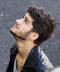Updated on 16-Jun-2020 14:12:48

1K+ Views

We know about the famous game Snake and Ladder. In this game, some rooms are present on the board, with the room number. Some rooms are connected with a ladder or with snakes. When we get a ladder, we can climb up to some rooms to reach near to the destination without moving sequentially. Similarly, when we get some snake, it sends us to a lower room to start the journey again from that room.In this problem, we have to find the minimum number of the dice throw is required to reach start to destination.Input and OutputInput: The starting and ... Read More

## Strongly Connected Graphs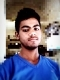Updated on 16-Jun-2020 14:16:40

2K+ Views

In a directed graph is said to be strongly connected, when there is a path between each pair of vertices in one component.To solve this algorithm, firstly, DFS algorithm is used to get the finish time of each vertex, now find the finish time of the transposed graph, then the vertices are sorted in descending order by topological sort.Input and OutputInput: Adjacency matrix of the graph. 0 0 1 1 0 1 0 0 0 0 0 1 0 0 0 0 0 0 0 1 0 0 0 0 0 Output: Following are strongly connected components in given ... Read More

## Shortest path with exactly k EdgesUpdated on 16-Jun-2020 12:34:11

299 Views

One directed graph is provided with the weight between each pair of vertices, and two vertices u and v are also provided. Our task is to find the shortest distance from vertex u to vertex v, with exactly k number of edges. To solve this problem, we will start from vertex u and go to all adjacent vertices and recur for adjacent vertices using the k value as k - 1.Input and OutputInput: The cost matrix of the graph. 0 10 3 2 ∞  0 ∞ 7 ∞  ∞ 0 6 ∞  ∞ ∞ 0 Output: Weight of the shortest ... Read More

## Maximum Bipartite MatchingUpdated on 16-Jun-2020 12:37:58

6K+ Views

The bipartite matching is a set of edges in a graph is chosen in such a way, that no two edges in that set will share an endpoint. The maximum matching is matching the maximum number of edges.When the maximum match is found, we cannot add another edge. If one edge is added to the maximum matched graph, it is no longer a matching. For a bipartite graph, there can be more than one maximum matching is possible.Input and OutputInput: The adjacency matrix. 0 1 1 0 0 0 1 0 0 1 0 0 0 0 1 0 0 ... Read More

## Shortest Path in a Directed Acyclic GraphUpdated on 16-Jun-2020 14:20:59

650 Views

One weighted directed acyclic graph is given. Another source vertex is also provided. Now we have to find the shortest distance from the starting node to all other vertices, in the graph.To detect Smaller distance, we can use another algorithm like Bellman-Ford for the graph with negative weight, for positive weight the Dijkstra’s algorithm is also helpful. Here for Directed Acyclic Graph, we will use the topological sorting technique to reduce complexity.Input and OutputInput: The cost matrix of the graph. 0   5  3 -∞ -∞ -∞ -∞  0  2  6 -∞ -∞ -∞ -∞  0  7  4  2 -∞ ... Read More

## How to find if a graph is Bipartite?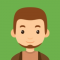Updated on 16-Jun-2020 12:41:36

4K+ Views

A graph is said to be a bipartite graph, when vertices of that graph can be divided into two independent sets such that every edge in the graph is either start from the first set and ended in the second set, or starts from the second set, connected to the first set, in other words, we can say that no edge can found in the same set.Checking of a bipartite graph is possible by using the vertex coloring. When a vertex is in the same set, it has the same color, for another set, the color will change.Input and OutputInput: ... Read More

## Longest Path in a Directed Acyclic GraphUpdated on 16-Jun-2020 12:46:03

933 Views

One weighted directed acyclic graph is given. Another source vertex is also provided. Now we have to find the longest distance from the starting node to all other vertices, in the graph.We need to sort the nodes in topological sorting technique, and the result after the topological sort is stored into a stack. After that repeatedly popped from the stack and try to find the longest distance for each vertex.Input and OutputInput: The cost matrix of the graph. 0  5   3 -∞ -∞ -∞ -∞ 0   2  6 -∞ -∞ -∞ -∞  0  7  4  2 -∞ -∞ ... Read More